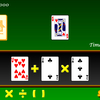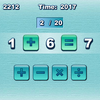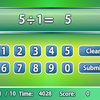# Smooth operators

Smooth Operators is a complete solution for learning, practicing, and testing the order of operations. An interactive lesson teaches the math concepts thoroughly with explanations and example problems, including an optional section on exponents. Popular memory aids are introduced, but users also learn a logical approach to truly understand the order of operations.

In practice mode, students click on operators in the correct order, giving their complete attention to which operations come first. In test mode, the user performs all calculations and types in the answers. Score is always displayed.

Problems can include parentheses and exponents, and the learner or supervisor can select the number of operations per problem and the size of operands. In the course of solving problems, users have an excellent review of basic math skills as they add, subtract, multiply, and divide positive and negative numbers.

Smooth Operators helps students master crucial skills and prepare for algebra and

## Related software (5)## Galactic Geometry 3D

Calculate volume and surface area to blast away rocks and save your space ship! Galactic Geometry is an engaging 3D environment for learning about geometric figures and their equat ...## Math Flight 2

Learning mathematics can be a challenge for anyone. Math Flight can help you master it with three fun activities to choose from! With lots of graphics and sound effects, your inter ...## Make 24

Use the given cards to form a valid equation within 60 seconds!## Quick Math

How quickly can you complete the equations with arithmetic symbols?